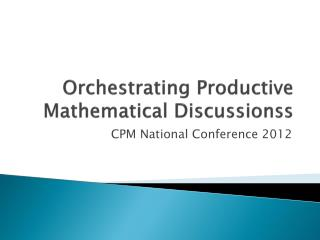Download PresentationOrchestrating Productive Mathematical DiscussionssOrchestrating Productive Mathematical Discussionss - PowerPoint PPT Presentation

Download PresentationOrchestrating Productive Mathematical Discussionss
An Image/Link below is provided (as is) to download presentation

Download Policy: Content on the Website is provided to you AS IS for your information and personal use and may not be sold / licensed / shared on other websites without getting consent from its author. While downloading, if for some reason you are not able to download a presentation, the publisher may have deleted the file from their server.

- - - - - - - - - - - - - - - - - - - - - - - - - - - E N D - - - - - - - - - - - - - - - - - - - - - - - - - - -
Presentation Transcript

1. Orchestrating Productive Mathematical Discussionss CPM National Conference 2012

2. Read and React “Ensuring that students have the opportunity to reason mathematically is one of the most difficult challenges that teachers face. A key component is creating a classroom in which discourse is encouraged and leads to better understanding. Productive discourse is not an accident, nor can it be accomplished by a teacher working on the fly, hoping for serendipitous student exchange that contains meaningful mathematical ideas.” Frederick Dillion

3. Think – Pair - Share • What do you do to plan a lesson? • To what extent does the cognitive demand of the lesson that you are using affect the level of planning in which you engage?

4. Lesson Structure • Lesson Opener • Explore – Students engaging in solving problems • Discuss and Summarize– This is the time in class when powerful learning and conversation occur. • Maintaining balance between the discipline of the mathematics and student authorship

5. Analyzing the case of David Crane • As you read the case, look for the balance between the mathematical goals of the lesson being clear and students authorship.

6. Five Practices • Anticipating • likely student responses to challenging mathematical tasks; • Monitoring • student’s actual responses to the tasks (while students work on the task in pairs or small groups); • Selecting • particular students to present their mathematical work during the whole-class discussion;

7. Sequencing • The student responses that will be displayed in a specific order; • Connecting • Different students’ responses and connecting the responses to key mathematical ideas

8. Foundation – Goal of lesson • Selecting the mathematical goal that is essential for every student should know and understand about math as a result of the lesson.

9. Rabbit Problem • Determine the learning goal for this lesson • Anticipate • Envision how students might mathematically approach this problem. • Record the various solution methods on the sample recording tool

10. In what sequence would you want the various solutions to occur? • What opportunities are there to make connections in order to highlight the mathematical goal?

11. Calling Plan Case Study • Mathematical Goals – Students should • Recognize that there is a point of intersection between two unique non parallel linear equations that represents where the two functions have the same x- and y- values • Understand that the two functions “switch positions” a the point of intersection • Make connections between the multiple representations and identify the slope and y-intercept in each representational form

12. Next Steps • How might I incorporate this into my practice? • What seems reasonable for how often I will use the Five practices in my planning? • Where do I want to start?

13. References 5 Practices for Orchestrating Mathematics Discussions by Margaret Smith & Mary Kay Stein Published by National Council of Teachers of Mathematics 2011